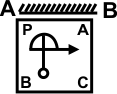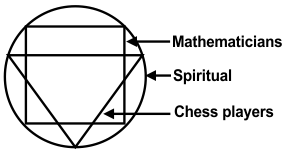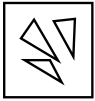﻿ Reasoning Olympiad (CRO) Previous Year Paper for Class 9

# CREST Reasoning Olympiad Class 9 Previous Year Papers

CREST Reasoning Olympiad Previous Year Paper PDF for Class 9:

## Syllabus:

Section 1: Series Completion, Analogy and Classification, Coding-Decoding, Blood Relations, Puzzle Test, Sequential Output Tracing , Direction Sense Test, Logical Venn Diagrams, Alphabet Test and Logical, Alpha-Numeric, Number, Mathematical Operations, Inserting the Missing Characters, Analytical Reasoning, Mirror Images and Water Images, Spotting Out The Embedded, Figure Matrix, Paper Folding and Paper Cutting, Grouping of Identical Figures, Cubes and Dice, Dot Situation, Construction of Squares and Triangles, Figure Formation and Analysis.

Achievers Section: Higher Order Thinking Questions - Syllabus as per Section 1

 Q.1 The post office is to the East of the school while my house is to the South of the school. The market is to the North of the post office. If the distance of the market from the post office is equal to the distance of my house from the school, in which direction is the market with respect to my school?
 Q.2 In the following question, choose the correct mirror image from the answer figures.Q.3 Read the following information carefully to answer the question given below it:I. 'A + B' means that 'A is the father of B'.II. 'A - B' means that 'A is the wife of B'.III. 'A x B' means that 'A is the brother of B'.IV. 'A ÷ B' means that 'A is the daughter of B'. If it is given 'P ÷ R + S + Q', then which of the following is true?
 Q.4 Study the given diagram carefully and spot out the false statement from among the four given options.Q.5 In the following question, choose which number completes the second pair in the same way as the first pair. 125 is to 62.5, as 8 is to _____________.
 Q.6 Six faces of a cube are painted as below: (i) Red opposite to black. (ii) Green in between red and black. (iii) Blue adjacent to white. (iv) Brown adjacent to blue.(v) Bottom face with red. Find the colour opposite to brown.
 Q.7 In the question given below, there are two statements followed by two conclusions numbered I and II. You have to take the two give statements to be true even if they seem to be at variance from commonly known facts and decide which of the given conclusion(s), logically follow(s) from the two given statements disregarding commonly known facts. StatementsI. Some desks are tables.II. Some tables are chairs. III. Some chairs are benches.IV. Some benches are cots.Conclusions I. Some chairs are desks.II. Some cots are tables.
 Q.8 In the following question, find the figure out of the answer choices figures, that can be formed by joining the pieces given in the question figure.Q.9 In the following question, one number is wrong in the series. Find out the number. 4, 19, 49, 93, 154, 229, 319
 Q.10 What should be the correct sign to balance the following equation? 17 - 3 × 6 = 45

 Q.1 b Q.2 c Q.3 c Q.4 c Q.5 c Q.6 a Q.7 d Q.8 c Q.9 a Q.10 d

Students can download the CREST Reasoning Olympiad previous year paper pdf for class 9 from this page. Each question in the paper carries 1 mark. The answer key for all the questions is also provided on the last page.

Class 9 CREST Reasoning Olympiad exam necessitates students to use logical thinking, analytical skills and much more. To prepare for the exam, Reasoning Olympiad previous year paper for class 9 will be very beneficial.

There are many advantages to practicing the Olympiad previous year paper as it provides students with a thorough understanding of the kind of questions they can anticipate in the test. Additionally, working through the CREST Reasoning Olympiad past year paper for class 9 will improve students' problem-solving abilities, which will be very helpful on test day.